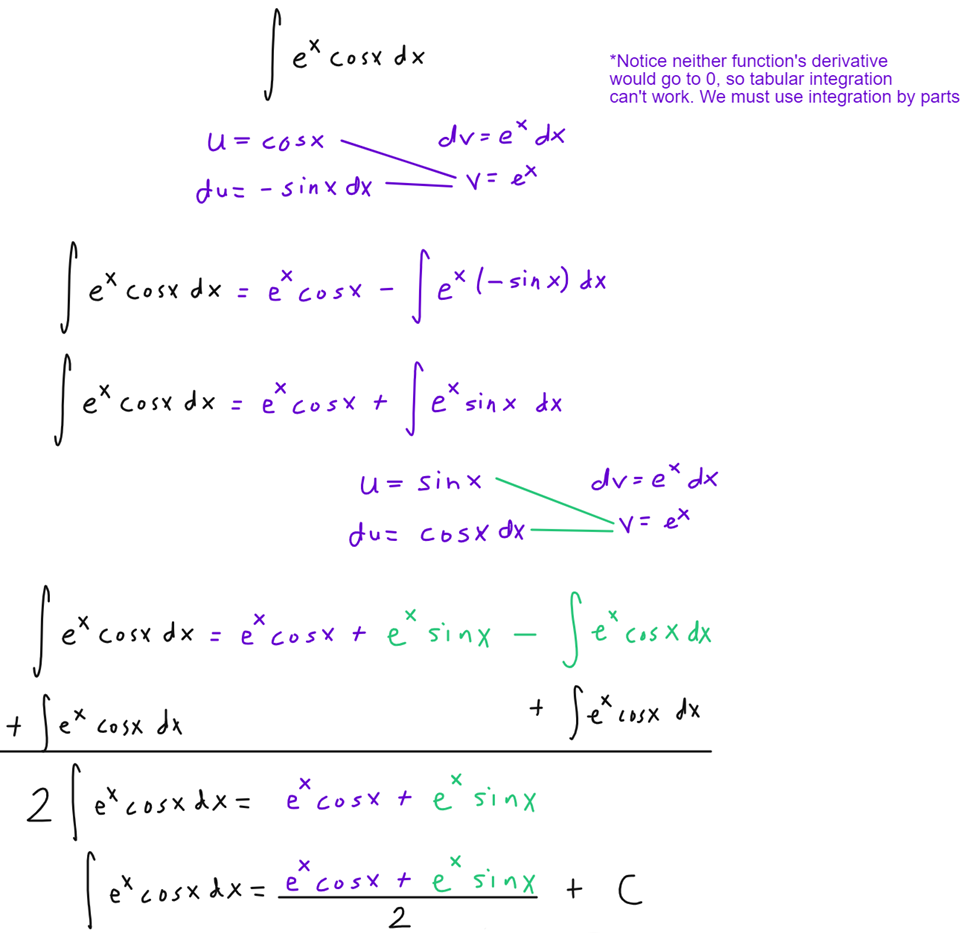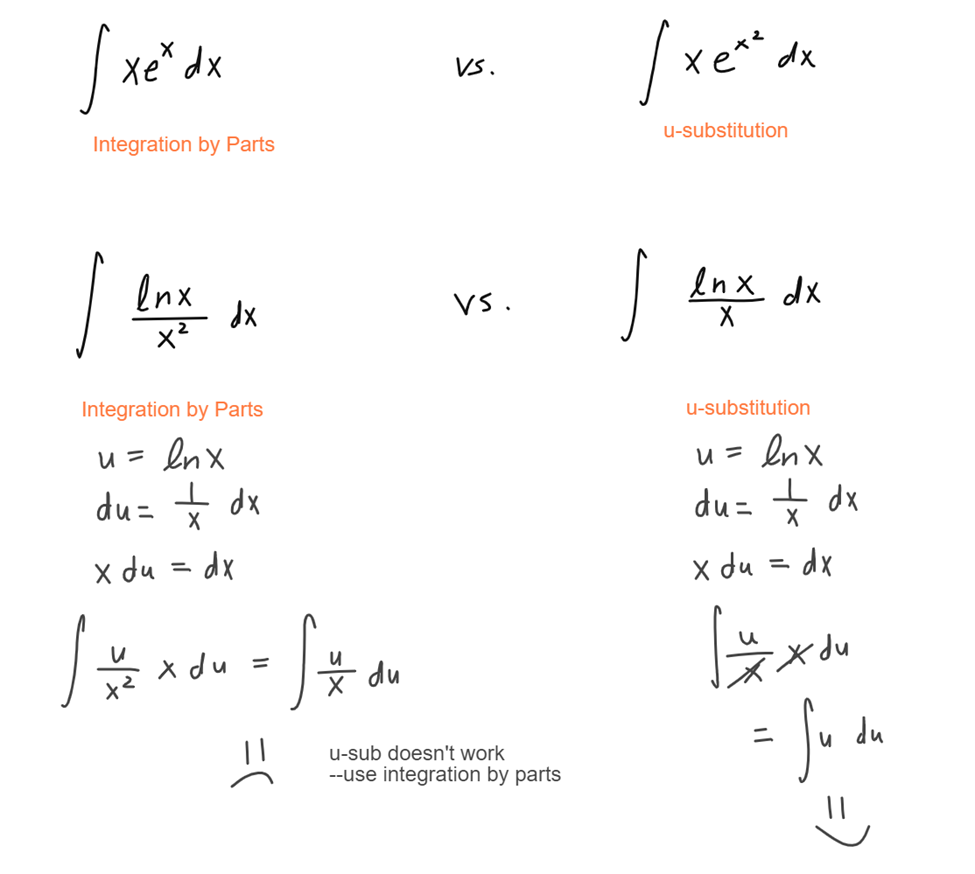top of page

# 5 Steps to Determine Which Integration Technique to Use

Updated: Sep 12

For Calculus students, determining which integration technique works is like figuring out which puzzle piece fits. This post will help you decide which method is appropriate for various integrals and how to check that your antiderivative is correct.

Especially in AP Calculus BC or Calculus II, students learn numerous integration techniques like u-substitution, integration by parts, partial fractions, and trigonometric substitutions. It may be easy to solve the homework questions where the methods are isolated, but when it comes to preparing for a test in which all the integration techniques are mixed, identifying the correct method gets challenging.

Below are the steps or sequence of questions I ask myself in order to determine which integration method to use. I’ll explain each method in more detail, providing examples, and common misconceptions at the end.AP Calculus AB or Calculus I students only cover the first three techniques, while AP Calculus BC or Calculus II students cover all five techniques.

## Which Integration Technique to Use

1. Is it a “basic” antiderivative?

Antiderivatives undo derivative rules. When I taught AP Calculus, I had students create notecards to help them memorize the derivative rules. Below is an illustration. Now, the notecards can be used in reverse to learn antiderivatives. Since the derivative of sin(x) is cos(x), the antiderivative of cos(x) is sin(x)!Here is a list of common derivatives and integrals that need to be memorized.

2. Can you rewrite the integrand (multiply, divide, rewrite exponents, trigonometric identities) to make it a basic antiderivative?

Below are examples of rewriting, separating the integrand into two terms or using exponent rules to rewrite radicals into exponents. Although you may see some trigonometric identities in homework problems, I wouldn’t worry about memorizing them for the AP exam. Having the derivative/antiderivative rules memorized is much more important!3. Is there a composition of functions? Use u-substitution.

A composition of functions is when you have functions f(x) and g(x), and the composition creates a new function h(x) = f(g(x)). This should look familiar as taking the derivative of a composition of functions uses the chain rule.

To undo the chain rule, or to take the antiderivative of a composition of functions, you want to use u-substitution. Set u to be the inside function. Based on the function h(x) above, u = g(x). Good choices for u would be the function inside parentheses, under a radical, in the denominator, or the exponent.

Two examples of u-substitution are below, but there is one specific nuance. If you have an integral with ln(x) or arctan(x), you must set u= ln(x) or u= arctan(x) because we don’t know their antiderivatives.There are two different approaches to rewriting the integral from being with respect to x to being with respect to u. The problem on the left I solved for dx and then replaced that for dx in the original integral. In the problem on the right, I looked for du in my integrand and had it exactly, so I didn’t need to multiply or divide by anything. Either way works so I wanted to provide both methods for you to determine which makes more sense to you, or which method your teacher uses.

As a side note of definitions before explaining the difference between the integrals in #3 and #4: A definite integral has bounds/limits at the bottom and top of the integral sign. This represents the area under the curve. An indefinite integral does not have bounds so the result is the family of curves which all have the same derivative, hence the +C at the end.

For the indefinite integral in #3, I plugged the u back in for the final step, so the answer is with respect to x. Notice for the definite integral in #4, however, I changed the bounds, so the new integral is all with respect to u. By changing everything to u, I don’t need to substitute lnx back in, although I see some students not switching the bounds and plugging u back in. You will get the same answer doing that, but changing the bounds is important as you may run into a question like from the 2012 AP Calculus exam:Without solving, I can tell at first glance that answer choices B and E are incorrect as the bounds did not change. The entire integral must be changed to be with respect to u, including the integrand, du, and the bounds/limits of integration.

4. Is there a product? Use integration by parts or tabular integration

If you have two functions being multiplied, a product, then integration by parts may be the best choice. Make sure you keep reading common misconceptions below, as some products are actually the result of a composition of functions and require u-substitution.

Integration by parts isn’t too long of a process but I love to take a shortcut if I can get the same result. So, I’ll use tabular integration whenever possible. Tabular integration works if one of the factors in the product has a derivative that eventually goes to 0. Specifically, if one of the factors is a polynomial, its derivative will go to 0 and tabular integration works well. See the example below using integration by parts on the left and tabular integration on the right.Notice why the shortcut of tabular integration works: multiplying diagonally is the same starting point from integration by parts u*v and then the signs alternate because we subtract the integral in integration by parts.

Many teachers suggest LIATE as a way to determine what to as set u for integration by parts. It’s an acronym for the functions: “Logarithmic,” “Inverse Trigonometric,” “Algebraic,” “Trigonometric,” and “Exponential.” In the example above, I set u=x, which is an algebraic function.

Sometimes you must do integration by parts multiple times, like in the example below. In that case, be consistent with your choice of u and dv. Since I used the trigonometric function as u, I stuck with that through both iterations of integration by parts.5. Is there a fraction whose denominator can be factored? Use partial fractions

If you don’t recall partial fraction decomposition from Pre-Calculus, see this article. Otherwise, check out the example problem below of how to integrate using partial fractions.Even when I get fractions for A and B, I put the entire fraction in the numerator. Notice I kept the ¾ in the numerator instead of 3 in the numerator and 4 in the denominator. This keeps the integral easier and avoids having to use u-substitution (see the very last example problem below).

Also, I rewrote the final answer as one logarithm. Recall from Pre-Calculus that you can condense two logarithms of the same base that are being subtracted into one logarithm dividing the arguments.

There are other integration techniques like trigonometric substitution, but this is not part of the AP Calculus BC curriculum. It is covered in most college Calculus II courses, so check out that process if it is covered in your Calculus course.

### Common Misconceptions

· Some products are actually compositions of functions, needing u-sub instead of integration by partsFor the first example, notice our normal function ex has an inside function of x2 for the problem on the right. That is a composition of functions so u-substitution should be used.

For the second example, it’s less obvious to use u-substitution for natural log. Recall that we always set u=lnx, whether we are using u-substitution or integration by parts. If you start a problem using u-substitution, a roadblock to know that u-sub doesn’t work is if the x terms don’t cancel out. We must be able to write our integral with respect to u only in order to use u-substitution.

· Some fractions are compositions of functions, using u-substitution, not partial fractions. For this scenario, set u to be the denominator

· Partial fractions may have hidden u-substitution in it if the derivative of the denominator isn’t 1Generally we’re used to jumping to the antiderivative being natural log of the denominator in partial fractions, but sometimes we have to account for the derivative of the denominator being something other than one.

Try out the steps above by practicing some antiderivatives! My post about the best resources to study for Calculus tests links numerous resources with practice problems.

If you’ve learned the Fundamental Theorem of Calculus, you know that derivatives and integrals are inverse operations. In other words, derivatives and integrals/antiderivatives undo each other. Therefore, you can check your answer by taking the derivative of your solution.

### Get Help Evaluating Integrals

Just like with a puzzle, some pieces look like they will fit until you try them out. Some integrals look like one method should work and it doesn’t, while others aren’t immediately obvious which method to use. Try out a method and you can always go back and restart with a different method if the first method doesn't work.

Are you still having trouble determining which method is correct for certain integrals? Join me in Calculus Crew or for individual tutoring and we can work through integration problems together!

bottom of page